My Favorite Number Theory Proof

Saturday, August 3, 2019, 9 AM – 11:20 AM, Duke Energy Convention Center, Rooms 207 & 208

Session Summary:

This session invites presenters to share favorite number theory proofs suitable for introduction to proofs courses or undergraduate number theory courses, but not graduate-level number theory courses. While non-standard proofs for the Fundamental Theorem of Arithmetic or the Chinese Remainder Theorem may be submitted for consideration, standard versions of these proofs and other typical proofs such as the irrationality of the square root of two, for example, will not be considered. Presenters must do the full proof, discuss how the proof fits into the course, provide information regarding prerequisite topics for the proof, and discuss associated areas with which students experience difficulty and how such concerns are addressed so that students understand the proof. Presenters are invited to discuss how they have modified the proof over time, share historical information related to “classic” proofs, and discuss explorations/demonstrations which they use to help students comprehend related theorems and topics. Abstracts should include the theorem to be proved/discussed as well as brief background information.

Summary for Abstracts Booklet:

Presenters share favorite proofs suitable for introductory proofs or undergraduate number theory courses, giving the complete proof, discussing how the proof fits into the course, providing information regarding prerequisite topics, areas of difficulty, and making the proof accessible for students. Modifications to the proof over time, historical information, and explorations/demonstrations used to make related theorems/topics comprehensible for students are discussed.

Organizer: Sarah L. Mabrouk, Framingham State University

 9 AM – 9:15 AM Divisibility, Modular Arithmetic, and Induction, Oh My! Martha H. Byrne, Sonoma State University For any natural number n, n3 – n is divisible by 6. Actually, this is true of any integer as well, and the proof techniques used don't change. It is well situated in an introduction to proofs course, as it can be proved in a variety of ways. Many novice proof writers struggle to find the “right” way to prove a claim to their detriment. In this talk, the presenter will go over several different proofs and talk about how to draw out student discussion about proof techniques that bridge the different approaches and support student understanding of multiple proving paths. 9:20 AM – 9:35 AM Various Teaching Strategies to Prove that a Certain Conjecture is Equivalent to Goldbach’s Conjecture Kristi Karber, University of Central Oklahoma One of the most famous conjectures in Number Theory authored by Goldbach states, “Every even integer greater than 2 can be expressed as the sum of two primes.” It can be shown that the conjecture, “Every integer greater than 5 is the sum of three primes,” is equivalent to Goldbach’s conjecture. In this talk we provide various strategies that can be used to help students understand or construct a proof which verifies the aforementioned conjectures are indeed equivalent. Possible strategies include an exploration, as well as an emphasis on beginning proof writing skills such as utilizing definitions and proof techniques. A proof will be provided while demonstrating the various approaches. 9:40 AM – 9:55 AM Fermat’s Bracelets and Wilson's Polygons: Seeing Two Foundational Theorems Geometrically Adam J. Hammett, Cedarville University Nearly every undergraduate course in number theory will include a presentation of both Fermat's little theorem and Wilson's theorem. Let p be a prime. The former states that for any given integer n > 0 we must have p | np – n, and the latter that p | (p – 1)! + 1. Most textbooks include proofs that, while elegant and brief, tend to labor in the realm of the purely analytical. However, George Andrews in his Number Theory text [pp. 36-40, Dover Publications, Inc., New York, 1994; MR0309838] gives remarkably simple, self-contained proofs of these results that rely only on symmetries of certain colored bracelets (in the case of Fermat) and special polygons (Wilson). In this talk, we will endeavor to present both of these gems in their entirety. 10 AM – 10:15 AM Euler’s Criterion Scott Williams, University of Central Oklahoma If a is an integer relatively prime to m, then we say a is a quadratic residue modulo m if a is a perfect square modulo m (i.e., if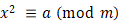has a solution). For p an odd prime, the Legendre symbol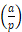takes a value of 1 if a is a quadratic residue modulo p and -1 otherwise. When initially exposed to quadratic residues, brute-force methods are generally what a student attempts to use to compute. Euler's Criterion gives them their first real computational approach to finding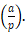Euler's Criterion:  Let p be an odd prime and let a be an integer not divisible by p.     Then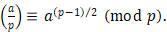One, possibly more standard, approach to proving Euler's Criterion involves the use of Fermat's Little Theorem as well as primitive roots. There is, however, an alternative proof which makes use of various other results discussed in an Elementary Number Theory course rather than primitive roots; e.g., the existence of modular inverses and Wilson's Theorem. In this talk we will discuss how the latter approach provides an excellent opportunity to tie together numerous concepts which are presented throughout such a course. 10:20 AM – 10:35 AM Seeding Polynomials for Quadratic Congruences Modulo Prime Powers Larry Lehman, University of Mary Washington The number of solutions of a quadratic congruence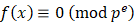can appear to be unpredictable when p2 divides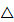, the discriminant of f. We will prove that these solutions can be counted and constructed in terms of those of a “seeding polynomial” for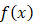, having discriminant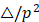. The proof is one that can be visualized, and is appropriate for a first course in number theory. As a consequence of this approach, we also establish a formula for the number of solutions of an arbitrary quadratic congruence modulo any prime power. 10:40 AM – 10:55 AM The Exact Power of p Dividing n! Scott Zinzer, Aurora University The Legendre formula gives the prime factorization of n!.  For a prime p, each factor of p appearing in n! must arise from a factor of p in one of the integers between 1 and n. The proof of the Legendre formula for p requires a careful counting of the number of integers between 1 and n that are divisible by each power of p. By using a measurement model for division on the number line, we demonstrate how the greatest integer function provides this count. We provide some initial motivation previewing why the sum of values of the greatest integer function successfully counts the exact number of times p appears as a factor among all of the integers between 1 and n. Finally, we use geometric sums to rewrite the Legendre formula for p in terms of the base-p expansion of n. 11 AM – 11:15 AM A Silver Version of Dirichlet's Bronze Approximation Theorem Andrew J. Simoson, King University Given a positive irrational number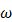and a reduced fractionof positive integers, we say thatis a bronze, silver, or gold approximation toif, respectively,and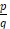differ by less than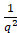,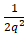, or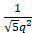. Johann Peter Gustav Lejeune Dirichlet proved that there are an infinite number of bronze winning fractions forin about 1840. Fifty years later, Adolf Hurwitz showed that there are an infinite number of gold winning fractions for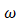. We shall do the same with respect to silver by using Farey sequences, named after John Farey (1766–1826), and Ford circles in the xy-plane, named after Lester R. Ford (1886–1967). Briefly, as follows from Euclid’s greatest common divisor algorithm, any reduced fractionis the mediant of two unique reduced fractionsand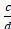, called Farey neighbors, where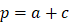and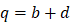. The Ford circle associated withis a circle with center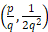and radius. A fun – and elementary – geometric argument shows that two Ford circles are tangent if and only if their associated fractions are Farey neighbors, which is enough to give us a recursion generating a sequence of only silver winning fractions for.

This page was created and is maintained by S. L. Mabrouk, Framingham State University.